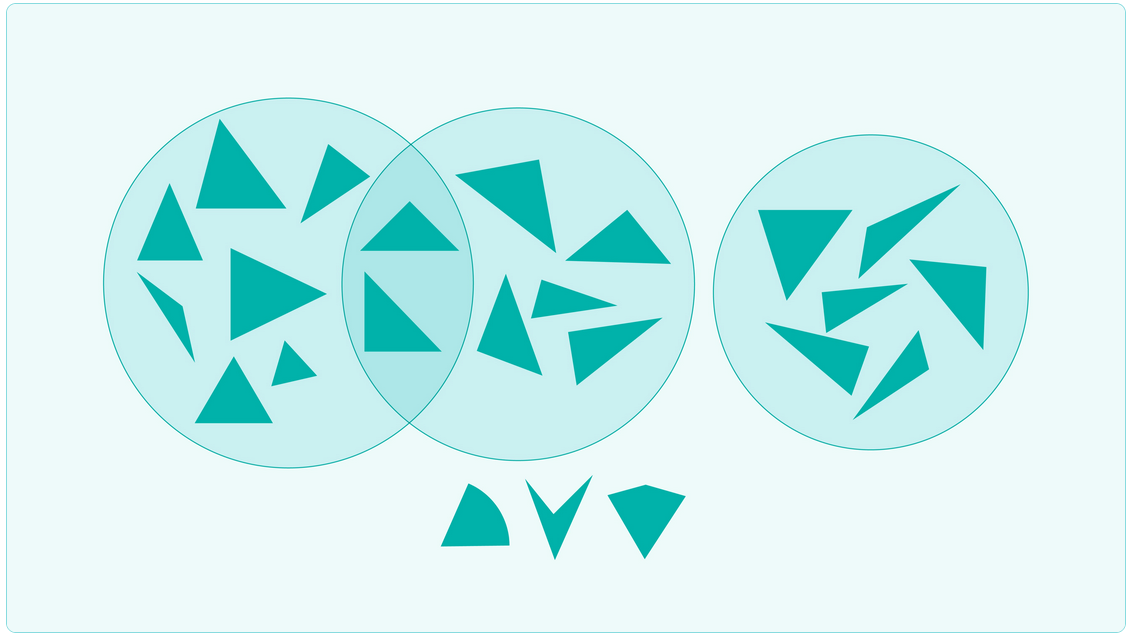# Mathematics level 4 - Measurement and geometry

## Teaching context - Geometric properties of shapes

Provide opportunities for students to compare and describe two-dimensional shapes and three-dimensional objects in terms of properties. Model the use of geometric language to support students' development of relevant vocabulary.

Students will demonstrate their learning by identifying and classifying two and three-dimensional objects.

They also explain their reasoning when recognising and describing the two-dimensional shapes that are the faces of three-dimensional objects. Provide students with the opportunity to use appropriate language to describe and sort shapes. The sorting activity should be followed up with a discussion where students justify why they have placed the shapes in certain sets and subsets.

Note some students' limited experience and exposure may have led to an over-reliance on commonly presented examples of various shapes, so they do not recognise other examples.

## Teaching idea - Sorting triangles

This activity allows students to choose criteria to make and justify classifications.

Students work in pairs or small groups and cut the shapes from Sheet T. The shapes on this sheet are mostly triangles and can be sorted into two or more sets according to criteria the students choose themselves, then into subsets according to other chosen criterion. A Venn diagram could be used for displaying the subsets of triangles.

Students at this level will be able to sort on two criteria at a time.

Possible criteria for sorting include:

• 0, 2 or 3 equal sides
• 0, 2 or 3 equal angles
• obtuse angle/no obtuse angle
• right angle/no right angle
• number of lines of symmetry (isosceles triangles have one and equilateral triangles have three)
• presence of rotational symmetry or not (equilateral triangles have one third turn symmetry).

The sorting activity should be followed up with an opportunity for students to justify why they have placed the shapes in certain sets and subsets. Guiding questions could include:

• 'Why do triangles have only one right angle?'
• 'How would you describe the number of lines of symmetry triangles can have?'
• 'Why can't a triangle have more than one obtuse angle?'
• 'What is the same and what is different about an equilateral triangle and an isosceles triangle?'## Extension ideas

### Sort triangles in to Venn diagrams

• Have students sort triangles into a three circle Venn diagram. They can come up with different solutions and justify their reasoning behind each. This provides high-ability students with the opportunity to explore the notion that shapes can be sorted in many different ways. It also allows these students to apply higher order thinking skills to the task.
• Give students different 3-D triangles to sort into either two or three circle Venn diagrams. Students could be included in the creation of these shapes using digital technologies. This adds complexity to the task for high-ability students as additional attributes must be considered in the decisions to sort the shapes.

### Explore optical illusions

Have students explore optical illusions that are created using triangles. For example, the impossible triangle. High-ability students can use the knowledge they gain about the properties of triangles to design their own optical illusion.

This task provides high-ability students with the option to explore the use of triangles in an artistic context. It gives them some control as they can decide on what illusions they explore. It requires high-ability students to engage in higher order levels of thinking as they analyse, evaluate, and then create.
This can be done individually or with like-ability peers, and students can share their solutions with others.

Original lesson plan available on the Maths Curriculum Companion